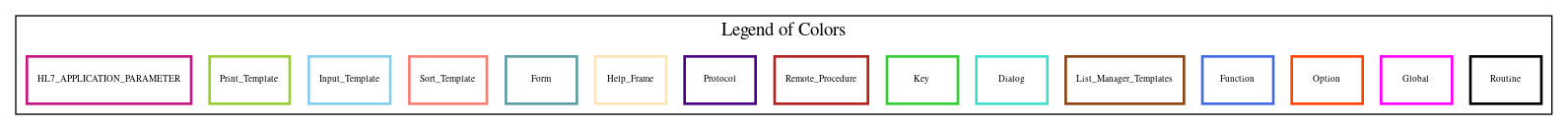Home   Package List   Routine Alphabetical List   Global Alphabetical List   FileMan Files List   FileMan Sub-Files List   Package Component Lists   Package-Namespace Mapping
Routine: ONCEDIT

Routine: ONCEDIT

Information

ONCEDIT ;Hines OIFO/GWB - Inter-field edits ;03/24/11

Source Information

Source file <ONCEDIT.m>

Call GraphCall Graph Total: 4

Package Total Call Graph
Oncology 2 ^ONCEDIT2  \$\$HIST^ONCFUNC
VA FileMan 2 \$\$GET1^DIQ  ^DIR

Caller Graph

Legends:Package Component Superscript legend

 action A extended action Ea event driver Ed subscriber Su protocol O limited protocol LP run routine RR broker B edit E server Se print P screenman SM inquire ICaller Graph Total: 2

Package Total Caller Graph
Oncology 2 ONCOAIS  ONCOEDC

Entry Points

IF02 ;S SDX=""
IF03
IF10
IF06
IF06EX
IF0708
IF11
IF11EX
FCTD
PHCSEQ
ERRMSG ;Error message
TXDT88 ;Treatment date = 88/88/8888 error message
CLEANUP ;Cleanup

External References

Name Field # of Occurrence
\$\$GET1^DIQ ONCEDIT+5, ONCEDIT+6, ONCEDIT+7, ONCEDIT+8, ONCEDIT+9, ONCEDIT+10, ONCEDIT+11, ONCEDIT+12, ONCEDIT+13, ONCEDIT+14
ONCEDIT+16, ONCEDIT+18, ONCEDIT+19, ONCEDIT+20, ONCEDIT+21, IF06+1, IF06+2, IF06+3, IF06+4, IF06+10
IF06+11, IF06+12, IF06+13, IF06+14, IF06+15, IF06+16, IF06+17, IF06+18, IF06+19, IF06+20
IF06+21, IF06+22, IF06+23, IF0708IF0708+1, IF0708+2, IF0708+3, IF0708+4, IF11+1, IF11+2
IF11EX+2, IF11EX+3, IF11EX+4, FCTD+1, PHCSEQPHCSEQ+1
^DIR IF0708+21
^ONCEDIT2 PHCSEQ+12
\$\$HIST^ONCFUNC ONCEDIT+15

Interaction Calls

Name Line Occurrences
Function Call: WRITE
• Prompt: !
• Line Location: IF0708+21
Function Call: WRITE
• Prompt: !," WARNING: "
• Line Location: ERRMSG+2
Function Call: WRITE
• Prompt: ?10,MSG(MSGSUB),!
• Line Location: ERRMSG+3
Routine Call
• DIR
• Line Location:
• IF0708+21

FileMan Files Accessed Via FileMan Db Call

FileNo Call Tags
^ONCO(164 - [#164] GET1^DIQ
^ONCO(165.5 - [#165.5] GET1^DIQ

Global Variables Directly Accessed

Name Line Occurrences  (* Changed,  ! Killed)
^ONCO(165.5 - [#165.5] IF0708+21*

Label References

Name Line Occurrences
ERRMSG IF03IF03+2, IF10IF06+24, IF06+26, IF06+28, IF06+30, IF06+32, IF06+34, IF06+36
IF0708+5, IF0708+9, IF0708+13, IF0708+16, IF0708+21, IF11+3, IF11+11, IF11+14, IF11EX+5, FCTD+2
FCTD+6, PHCSEQ+6
IF06EX IF06+5
IF11 IF0708+20
IF11EX IF11

Local Variables

Legend:

 >> Not killed explicitly * Changed ! Killed ~ Newed

Name Field # of Occurrence
BCOD ONCEDIT+3~, ONCEDIT+17*
BRME IF06+21*, IF06+35, IF06EX!
BRMI IF06+20*, IF06+34, IF06EX!
CHME IF06+17*, IF06+31, IF06EX!
CHMI IF06+16*, IF06+30, IF06EX!
CMPLT IF0708+21*, IF0708+22, ERRMSG+1*, CLEANUP+1!
CNSE IF06+15*, IF06+29, IF06EX!
CNSI IF06+14*, IF06+28, IF06EX!
COCE ONCEDIT+3~, ONCEDIT+7*, IF10+2, IF06+6, IF0708+11
COCI ONCEDIT+3~, ONCEDIT+6*, IF10IF10+1, IF06+9, IF0708+5, IF0708+9, IF0708+10, FCTD+2, FCTD+6
DDXE IF06~, IF06+4*
DDXI IF06~, IF06+3*, IF06+28, IF11+3
DIAE IF06+2*, IF06+5, IF06+7, IF06EX!
DIAI IF06+1*, IF06+24, IF06+26, IF06+28, IF06+30, IF06+32, IF06+34, IF06+36, IF06EX!
DIR IF0708+21!, IF0708+26!
DIR("A" IF0708+21*
DIR("B" IF0708+21*
DIR(0 IF0708+21*
>> DTDX ONCEDIT+18, ONCEDIT+19, ONCEDIT+20, ONCEDIT+21
DXCF IF0708*, IF0708+13, IF0708+16, IF0708+21, IF0708+23, IF0708+26!
FCTD FCTD~, FCTD+1*, FCTD+2, FCTD+6
HORE IF06+19*, IF06+33, IF06EX!
HORI IF06+18*, IF06+32, IF06EX!
HSTE ONCEDIT+3~, ONCEDIT+16*
HSTFLD ONCEDIT+3~, ONCEDIT+15, ONCEDIT+16
HSTI ONCEDIT+3~, ONCEDIT+15*, ONCEDIT+17
LRTI IF11EX+4*, IF11EX+5, IF11EX+8!
LTRL IF11+2*, IF11+3, IF11+11, IF11+14, IF11EX!
MSG ONCEDIT+4!, IF10+4!, IF06EX!, IF0708+26!, IF11EX!, IF11EX+8!, PHCSEQ+10!
MSG( ERRMSG+3
MSG(1 IF03+1*, IF03+3*, IF10+1*, IF06+6*, IF0708+6*, IF0708+10*, IF0708+14*, IF0708+17*, IF0708+23*, IF11+4*
IF11+12*, IF11+15*, IF11EX+6*, FCTD+3*, FCTD+7*, PHCSEQ+7*
MSG(2 IF10+2*, IF06+7*, IF0708+7*, IF0708+11*, IF0708+15*, IF0708+18*, IF0708+24*, IF11+5*, IF11+13*, IF11+16*
IF11EX+7*, FCTD+4*, FCTD+8*, PHCSEQ+8*, TXDT88+1*
MSG(3 IF10+3*, IF06+8*, IF0708+8*, IF0708+12*, IF0708+19*, IF0708+25*, IF11+6*, FCTD+5*, FCTD+9*, PHCSEQ+9*
TXDT88+2*
MSG(4 IF06+25*, IF06+27*, IF06+29*, IF06+31*, IF06+33*, IF06+35*, IF06+37*, IF11+7*, IF11+8*, IF11+9*
IF11+10*
MSGSUB ERRMSG+3*, ERRMSG+5!
OTHE IF06+23*, IF06+37, IF06EX!
OTHI IF06+22*, IF06+36, IF06EX+1!
OVER IF0708+4*, IF0708+21, IF0708+26!
OVERRIDE TXDT88+3*, CLEANUP+1!
PHC PHCSEQ+1*, PHCSEQ+6, PHCSEQ+10!
PORG IF11+1*, IF11+3, IF11+11, IF11+14, IF11EX!
PRIM IF0708+3*, IF0708+20, IF0708+26!
PRM ONCEDIT+5, ONCEDIT+6, ONCEDIT+7, ONCEDIT+8, ONCEDIT+9, ONCEDIT+10, ONCEDIT+11, ONCEDIT+12, ONCEDIT+13, ONCEDIT+14
ONCEDIT+15, ONCEDIT+16, ONCEDIT+18, ONCEDIT+19, ONCEDIT+20, ONCEDIT+21, IF06+1, IF06+2, IF06+3, IF06+4
IF06+10, IF06+11, IF06+12, IF06+13, IF06+14, IF06+15, IF06+16, IF06+17, IF06+18, IF06+19
IF06+20, IF06+21, IF06+22, IF06+23, IF0708IF0708+1, IF0708+2, IF0708+3, IF0708+4, IF0708+21
IF11+2, IF11EX+2, IF11EX+3, IF11EX+4, FCTD+1, PHCSEQPHCSEQ+1, CLEANUP+1!
RADE IF06+13*, IF06+27, IF06EX+1!, IF11EX+3*, IF11EX+6, IF11EX+8!
RADI IF06+12*, IF06+26, IF06EX+1!, IF11EX+2*, IF11EX+5, IF11EX+6, IF11EX+8!
RFAC ONCEDIT+11*, IF03IF10IF10+4!
RHSP ONCEDIT+5*, IF03IF03+2, IF10+4!
SAVCMPLT IF0708+21, IF0708+22*, IF0708+26!
SEQ IF0708+1*, IF0708+24, IF0708+26!, PHCSEQ*, PHCSEQ+6, PHCSEQ+8, PHCSEQ+10!
SEQN IF0708+2*, IF0708+21, IF0708+26!
SPSE IF06+11*, IF06+25, IF06EX+1!
SPSI IF06+10*, IF06+24, IF06EX+1!
SSTE ONCEDIT+3~, ONCEDIT+19*, ONCEDIT+21*
SSTI ONCEDIT+3~, ONCEDIT+18*, ONCEDIT+20*
STAT ONCEDIT+3~, ONCEDIT+8*
TFAC ONCEDIT+12*, IF03+2, IF10+4!
TOPE ONCEDIT+3~, ONCEDIT+14*, IF11+4, IF11+12, IF11+15
TOPI ONCEDIT+3~, ONCEDIT+13*, IF11IF11+1, IF11+7, IF11+8, IF11+9, IF11+10
TRSE ONCEDIT+3~, ONCEDIT+10*, IF0708+6, IF0708+14, IF0708+17
TRSI ONCEDIT+3~, ONCEDIT+9*, IF0708+5, IF0708+9, IF0708+13, IF0708+16
U IF0708+21
X ONCEDIT+3~
Y IF0708+21, IF0708+26!
Z ERRMSG+4*, ERRMSG+5!